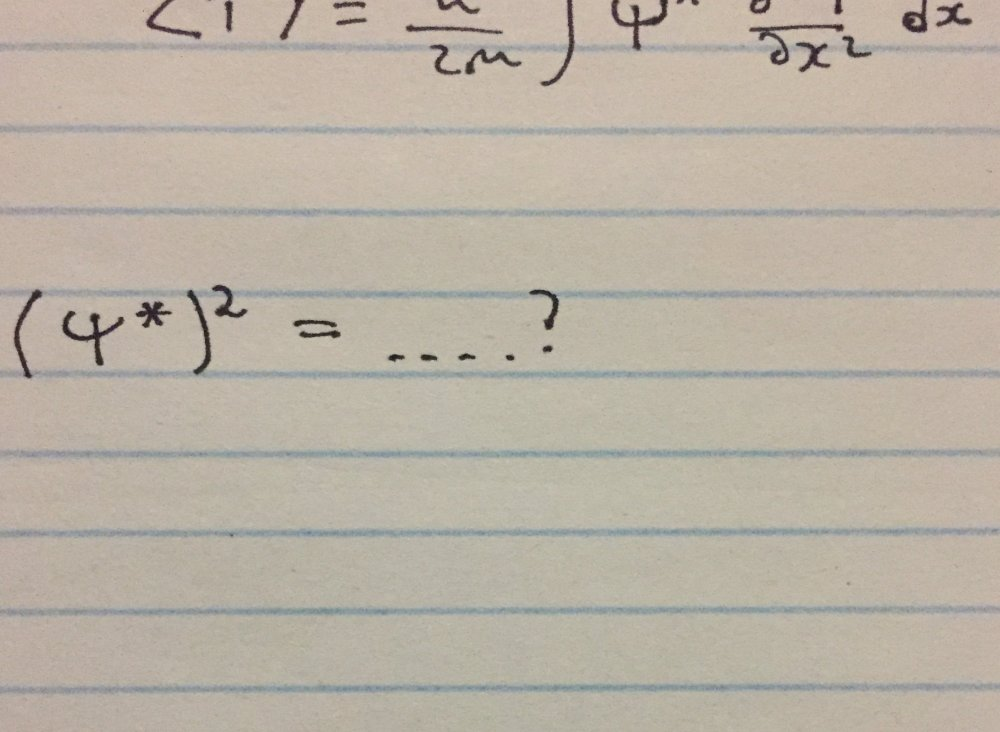## Recommended Posts

I was computing an expectation value of kinetic energy.

Here the kinetic energy is the square of momentum divided by 2m.

But when I square expectation value of momentum, the textbook return Psi unsquared##### Share on other sites
Posted (edited)

Your image cut off the top equation. Here is a hint the purpose to square the expectation value is to return a positive norm. All probability functions and energy densities are positive norm.

Edited by Mordred

## Create an account

Register a new account